Levels »

# Feel The Power

### The Catacombs, Elevation: -2.4m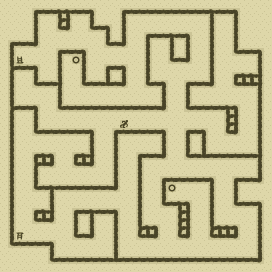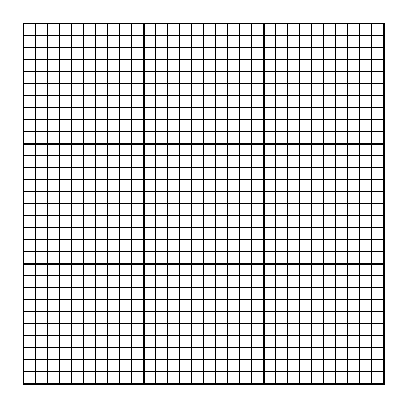### Items

•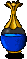Clear Blue Potion (30, 6)
•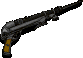Rusted MP-41 (14, 14) (on Walter)
•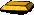Gold Ingot (13, 13)
•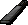7 MP-41 Magazines (218 rounds) (27, 1) x32 - (1, 8) x32 - (14, 14) x26 (in Rusted MP-41) (on Walter) - (14, 14) x32 (on Walter) - (14, 14) x32 (on Walter) - (14, 14) x32 (on Walter) - (15, 14) x32

•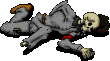(14, 14) Walter
•Rusted MP-41
•MP-41 Magazine x26
•MP-41 Magazine x32
•MP-41 Magazine x32
•MP-41 Magazine x32

### Monsters

•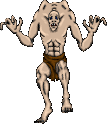4 Ghouls (Random rate: 2) (16, 2) - (30, 13) - (12, 14) - (18, 20)
•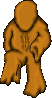45 Oozes (Random rate: 6) (4, 1) - (26, 1) - (27, 1) - (4, 2) - (5, 2) - (26, 2) - (18, 4) - (19, 4) - (1, 5) - (2, 5) - (19, 5) - (2, 6) - (7, 6) - (8, 6) - (7, 7) - (1, 8) - (2, 8) - (1, 9) - (1, 13) - (2, 13) - (20, 13) - (21, 13) - (2, 14) - (20, 14) - (17, 16) - (18, 16) - (18, 17) - (29, 19) - (30, 19) - (4, 20) - (30, 20) - (4, 21) - (5, 21) - (20, 22) - (4, 23) - (20, 23) - (21, 23) - (3, 24) - (4, 24) - (6, 25) - (6, 26) - (7, 26) - (11, 26) - (11, 27) - (12, 27)

### Locations

• 2 Save runes(8, 6) - (20, 22)